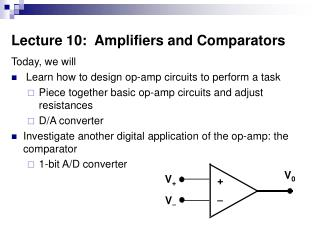DownloadDownload PresentationLecture 10: Amplifiers and Comparators

# Lecture 10: Amplifiers and Comparators

Download Presentation## Lecture 10: Amplifiers and Comparators

- - - - - - - - - - - - - - - - - - - - - - - - - - - E N D - - - - - - - - - - - - - - - - - - - - - - - - - - -
##### Presentation Transcript

1. V0 V+ +  V Lecture 10: Amplifiers and Comparators Today, we will • Learn how to design op-amp circuits to perform a task • Piece together basic op-amp circuits and adjust resistances • D/A converter • Investigate another digital application of the op-amp: the comparator • 1-bit A/D converter

2. Designing Op-Amp Circuits • You can design a new op-amp circuit by connecting our basic op-amp circuits together and selecting resistor values. • Even if there is an element (or another circuit) attached to the output of an op-amp circuit, the op-amp circuit behaves the same. • Break the desired task into smaller pieces which are easily done with one op-amp circuit, then connect the circuits together.

3. Example • Design a circuit whose output is:

4. Number Representation • A computer represents the number “1” (logic 1) by some positive voltage; usually 3 V to 5 V. • The number “0” is represented by 0 V. • A number in the computer is stored in binary, or base two representation. • Each binary digit (bit) is represented by a voltage at a separate point. V2 Memory Chip V1 3-bit memory V0

5. Number Representation • In the circuit below, V0 could represent the “ones” place, V1 the “twos” place, and V2 the “fours” place. • One can have either a 1 or a 0 in each place. So, the 3-bit memory can store numbers 0 through 7. • The number in each place is represented by a voltage, 0 V for 0 and, say, 5 V for 1. V2 Memory Chip V1 3-bit memory V0

6. Digital to Analog (D/A) Conversion • The op-amp circuit that we just designed converts the digital number representation in our memory chip to an analog representation. • It takes a number currently represented by three voltages with place values, and reinterprets the number so that “1” is represented by 1 V, “6” is represented by 6 V, etc. The circuit: • Divides each input voltage by 5 so each will have the value 0 V or 1 V • Multiplies by the place value that number represents • Adds up the numbers

7. D/A Conversion • Your stereo speaker has cones in it that vibrate to make the sound. An analog voltage causes the cones to vibrate. • The D/A converter helps translate digitally stored music into an analog voltage for the speakers. • Digital music (CD, MP3) provides a number indicating the sound amplitude at each sample time. These numbers get translated into analog voltage by the D/A converter. • The more bits used to store each sample, the more audio levels represented (better quality)

8. A/D Conversion, Signal Degradation • Naturally, we want to be able to go in the other direction as well, and convert analog representation to digital. • This is useful not only in audio and data acquisition, but within digital computation as well. • As a digital signal propagates, it is degraded by natural resistance and capacitance in circuits. • Pretty soon, the signal is not only 0 or 1 most of the time, but has in-between (nonsense) voltages too. Always 0 or 1 Degraded Signal

9. Comparator The degraded signal can be “cleaned up”, transformed into a signal which is nearly always 0 or 1, using a comparator. To make a comparator, • We set the high rail on the op-amp to the logic 1 voltage, and the low rail to logic 0 (0 V). • We set the threshold voltage VTHR to be around halfway between logic 0 and logic 1. V0 VIN + 

10. V0 VIN +  Using the Rails • Note that in the linear region, VO = A (VIN – VTHR). • Since A is large, the amplifier will hit the top rail when VIN is just a little above VTHR. • It will hit the low rail when VIN is just a little lower than VTHR. • Only a small range of VIN will leave it in the linear region. • The comparator “decides” whether VIN is logic 0 or logic 1. V0 Slope is A

11. Example • Suppose we have a comparator with: • Logic 1 voltage = 5 V • Logic 0 voltage = 0 V • Threshold voltage = 2 V • Ri = ∞, RO = 0 Ω, A = 1000 • For the input signal VIN(t) = 5 – e-2t V, sketch VO(t) for t between 0 and 3 seconds.

12. 2-Bit A/D Converter (with rounding)